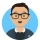Home
IT Knowledge
Inspiration
Languages
EN

# JavaScript - how to find max value in array with Array reduce method?

9 points
Created by:Krzysiek
351

ECMAScript 2009 introduced `Array` `reduce()` method that helps to transform array to different object doing some operations on each one element. As one usage case we can find maximum value in array, that is verry useful when we want to compare items by some nested properties.

## 1. ES 6 and newests example

In ECMAScript 2015 we can use arrow functions that makes code more clear.

``````// ONLINE-RUNNER:browser;

const array = [1, 2, 3];
const result = array.reduce((a, b) => Math.max(a, b));

console.log(result);``````

With nested properties:

``````// ONLINE-RUNNER:browser;

const array = [{value: 1}, {value: 2}, {value: 3}];
const result = array.reduce((r, b) => Math.max(r, b.value), Number.NEGATIVE_INFINITY);

console.log(result);``````

## 2. ES 5 example

In ECMAScript 2009 we need to use classic function notation.

``````// ONLINE-RUNNER:browser;

var array = [1, 2, 3];

var result = array.reduce(function(a, b) {
return Math.max(a, b);
});

console.log(result);``````